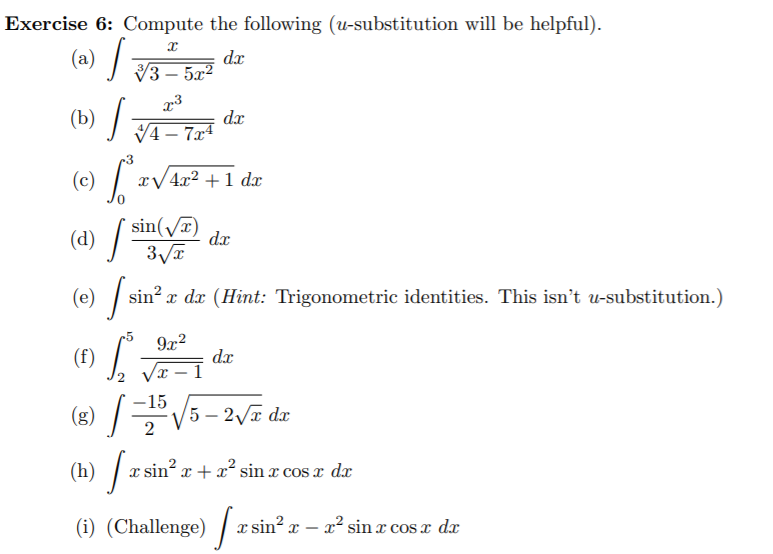# Exercise 6: Compute the following (u-substitution will be helpful).(a) /dxV3 – 5a?- 5x²(b) /dxV4 – 7x4-3(c) / :xV4x2 +1 dxsin(/T)dx(d) /3VT(0) /srsin? x dx (Hint: Trigonometric identities. This isn't u-substitution.)-59x2(f)dxVx – I-15(8) V5 – 27 dx(h) / a:sin? x + x sin x cos x d(i) (Challenge) /T. sin? x –x² sin x cosx dx

Question
2 views

subparts d,e,fhelp_outlineImage TranscriptioncloseExercise 6: Compute the following (u-substitution will be helpful). (a) / dx V3 – 5a? - 5x² (b) / dx V4 – 7x4 -3 (c) / : xV4x2 +1 dx sin(/T) dx (d) / 3VT (0) /sr sin? x dx (Hint: Trigonometric identities. This isn't u-substitution.) -5 9x2 (f) dx Vx – I -15 (8) V5 – 27 dx (h) / a: sin? x + x sin x cos x d (i) (Challenge) / T. sin? x – x² sin x cosx dx fullscreen
check_circle

Step 1
1. d) Given integral is,
Step 2
Step 3

### Want to see the full answer?

See Solution

#### Want to see this answer and more?

Solutions are written by subject experts who are available 24/7. Questions are typically answered within 1 hour.*

See Solution
*Response times may vary by subject and question.
Tagged in

### Integration